# 爆肝万字，终于搞定这篇⛵神经网络搭建全全全流程！学不会你来找我~

+关注继续查看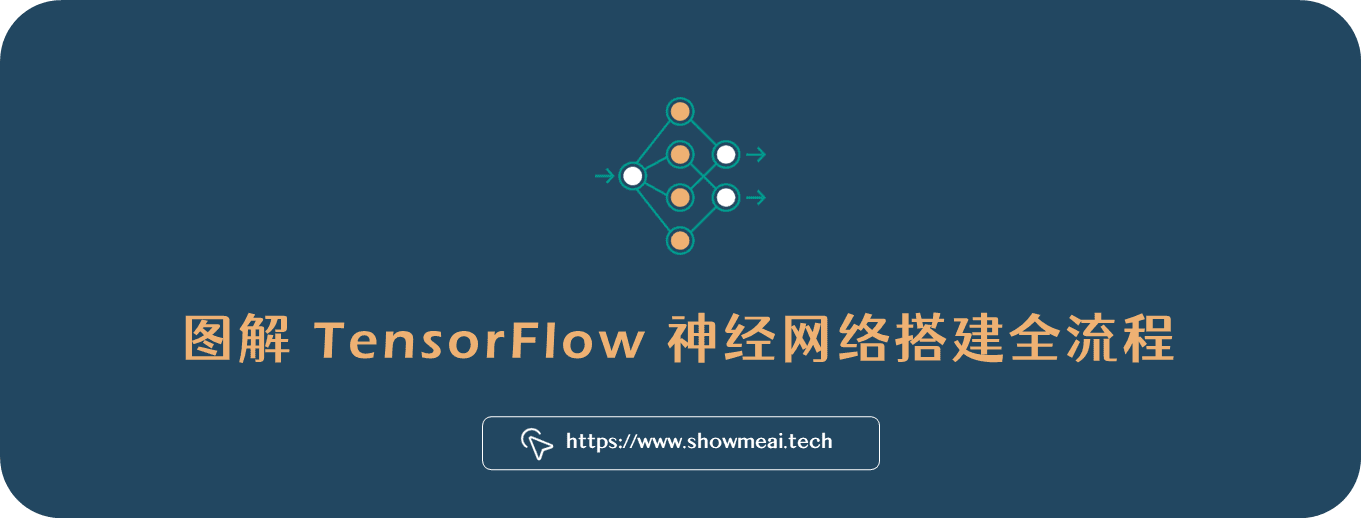💡 作者：韩信子@ShowMeAI
📘 深度学习实战系列https://www.showmeai.tech/tutorials/42
📘 TensorFlow实战系列: https://www.showmeai.tech/tutorials/43
📘 本文地址https://www.showmeai.tech/article-detail/290
📢 声明：版权所有，转载请联系平台与作者并注明出处
📢 收藏ShowMeAI查看更多精彩内容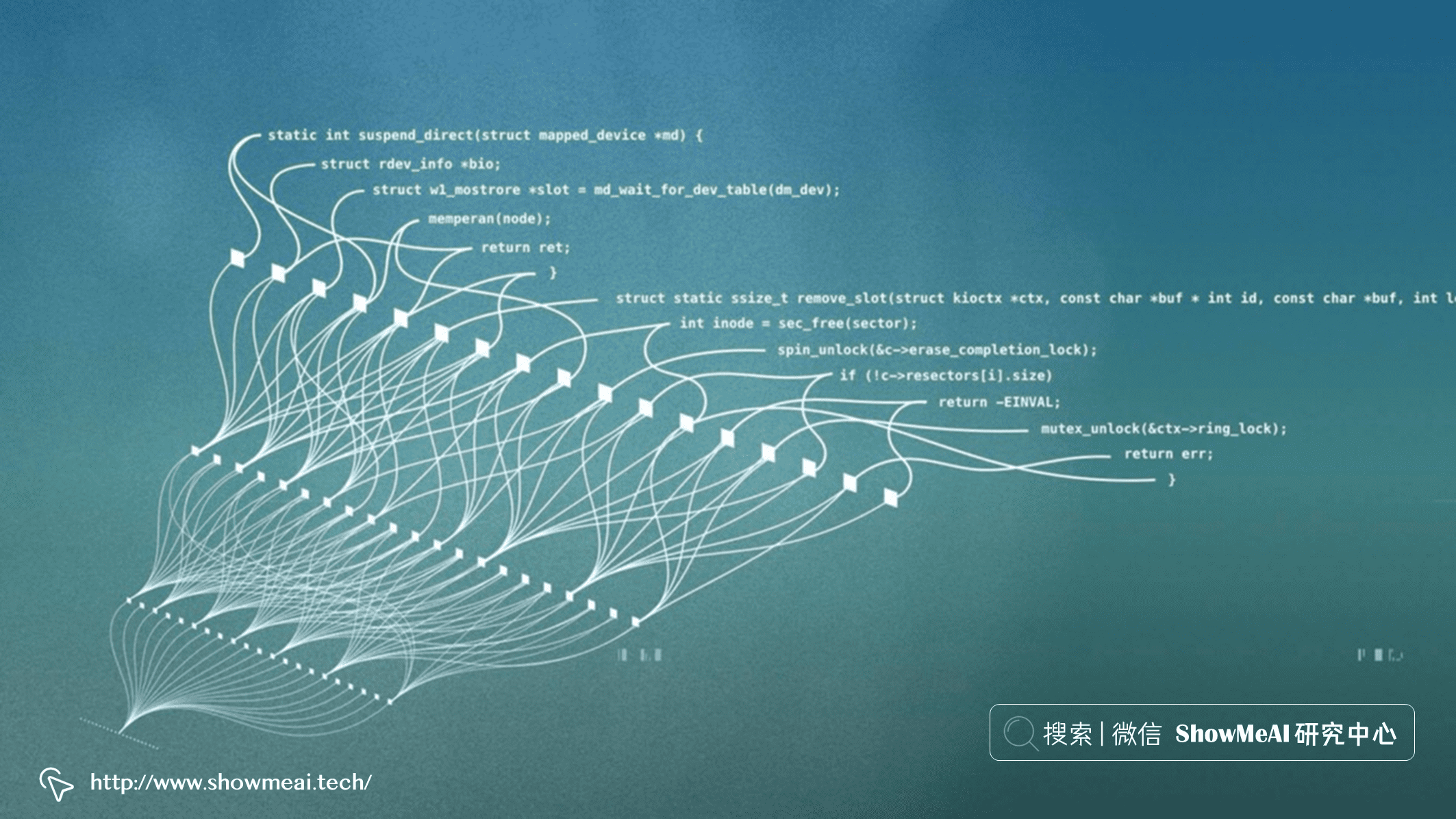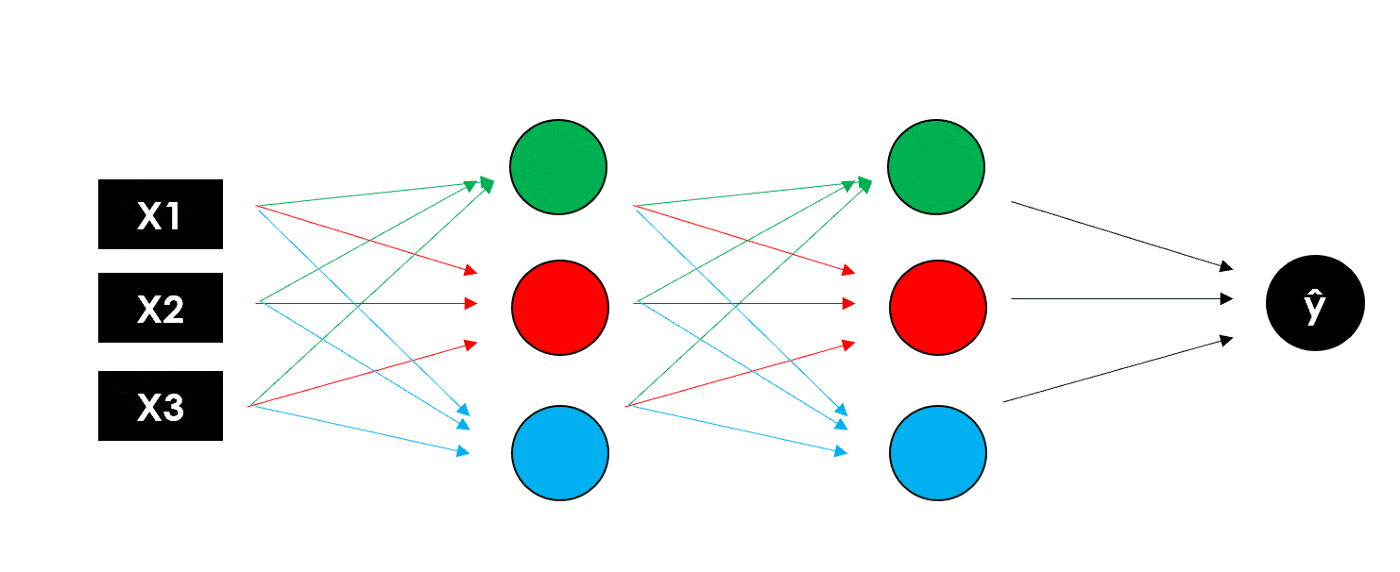ShowMeAI 将在本文中，全面图解展示使用 Python 构建神经网络的过程，覆盖TensorFlow建模、可视化网络、模型预测结果归因与解释。

• 环境设置与TensorFlow工具库简介
• 神经网络分解、输入、输出、隐藏层、激活函数
• 使用深度神经网络进行深度学习
• 进行模型设计（基于TensorFlow）
• 可视化神经网络
• 模型训练和测试
• 模型可解释性

# 💡 环境设置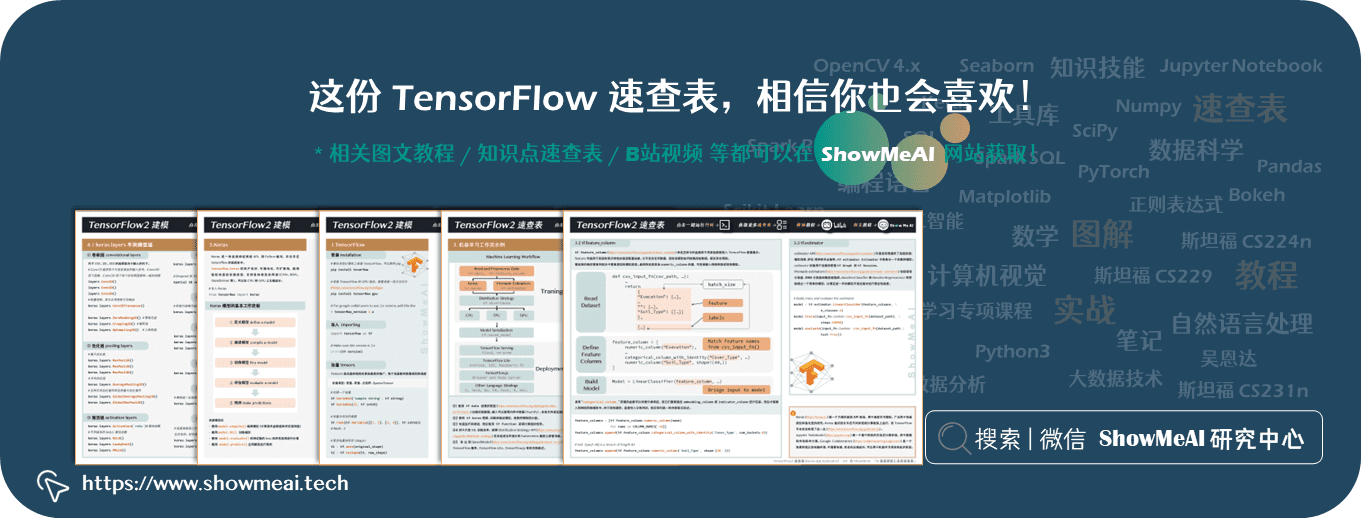pip install tensorflow

# 导入所需的工具库
# tensorflow建模
from tensorflow.keras import models, layers, utils, backend as K
# 可视化
import matplotlib.pyplot as plt
# 特征重要度与模型归因分析
import shap

# 💡 神经网络拆解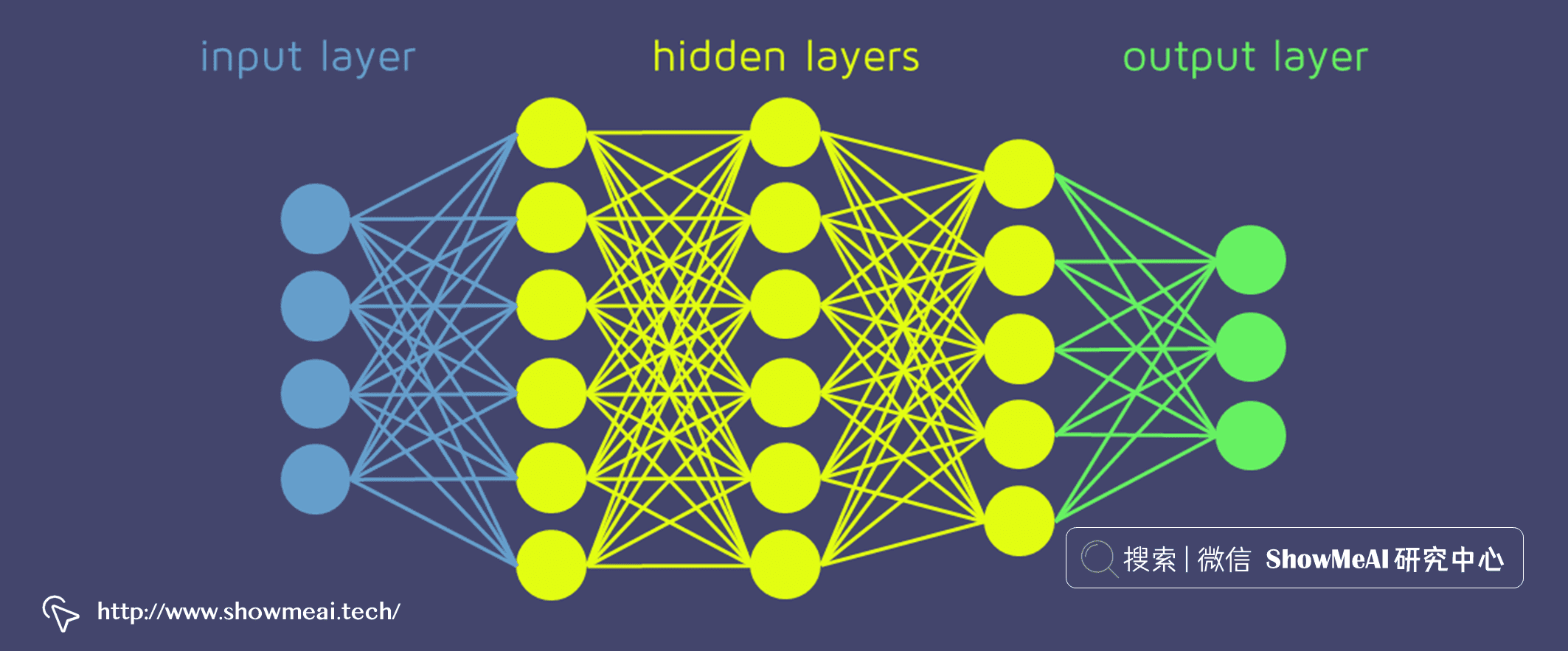• 输入层 负责将输入向量传递给神经网络。如果我们有一个包含 3 个特征的矩阵（形状 N x 3），则该层将 3 个数字作为输入，并将相同的 3 个数字传递给下一层。
• 隐藏层 代表中间节点，它们对数字进行多次变换以提高最终结果的准确性，输出由神经元的数量定义。
• 输出层 返回神经网络最终输出的 如果我们进行简单的二元分类或回归，输出层应该只有 1 个神经元（因此它只返回 1 个数字）。在具有 5 个不同类别的多类别分类的情况下，输出层应有 5 个神经元。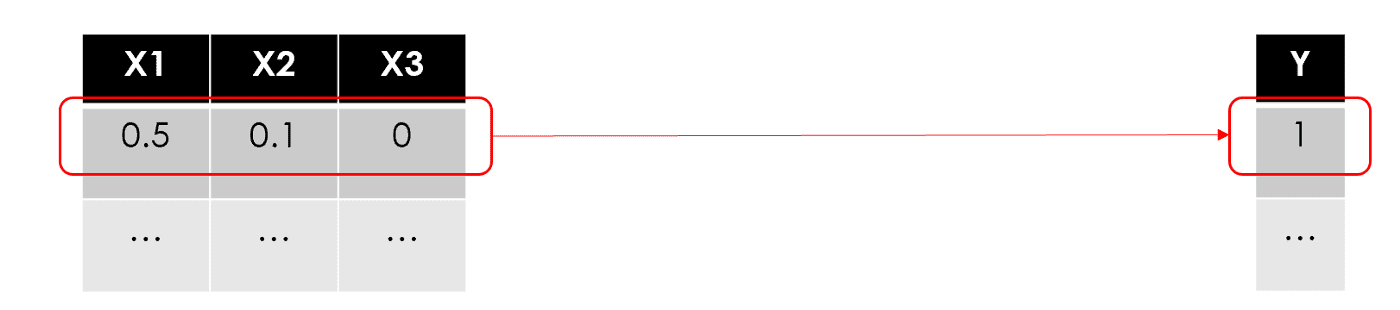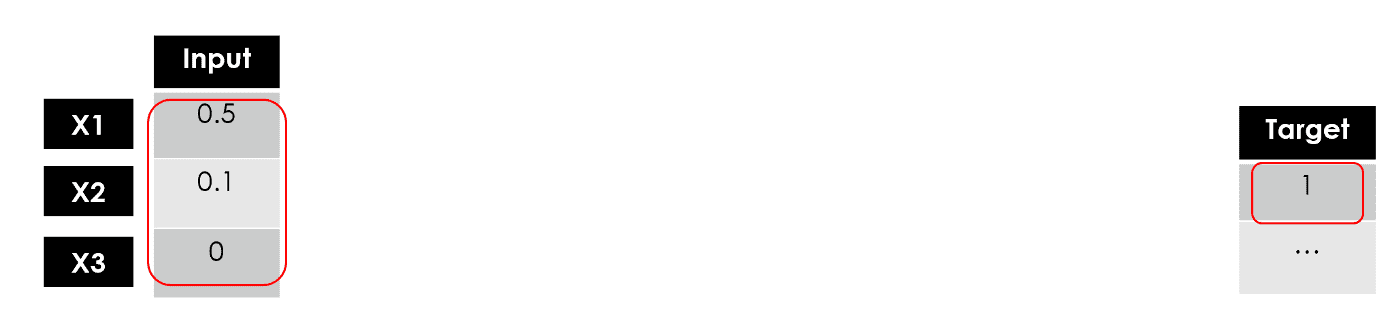• 在线性回归中，是找到最佳权重w
• 在基于树的模型（比如随机森林）中，它是找到最佳分裂点
• 如下的感知器中，我们希望找到最佳的W（w1，w2，w3）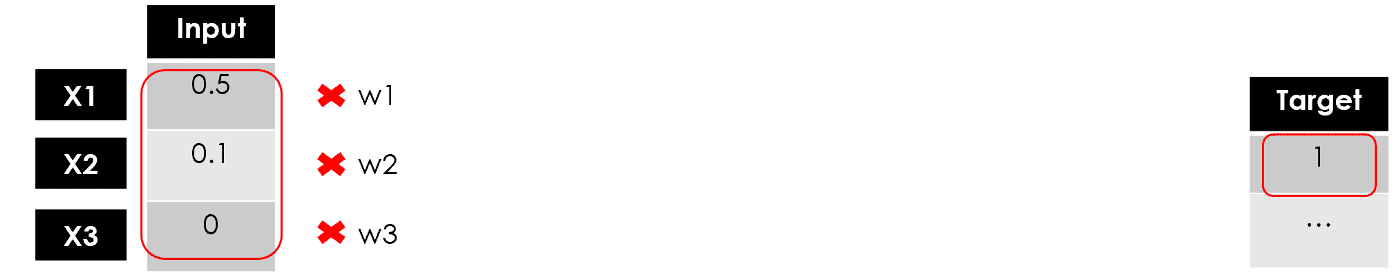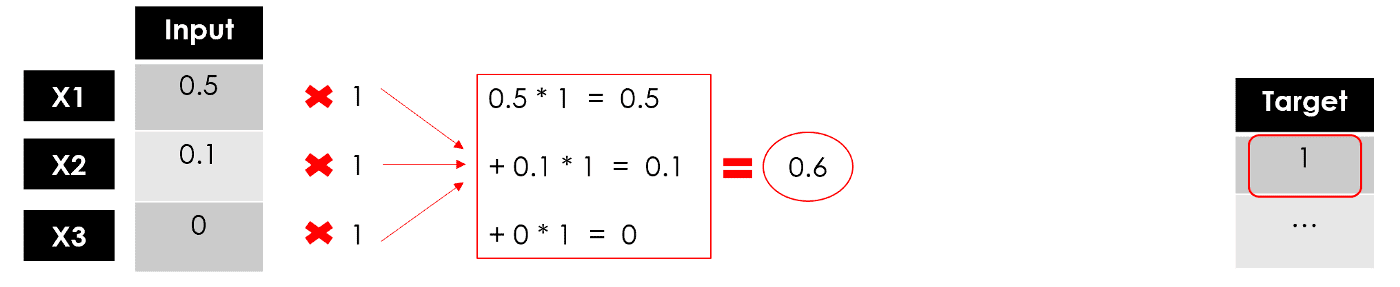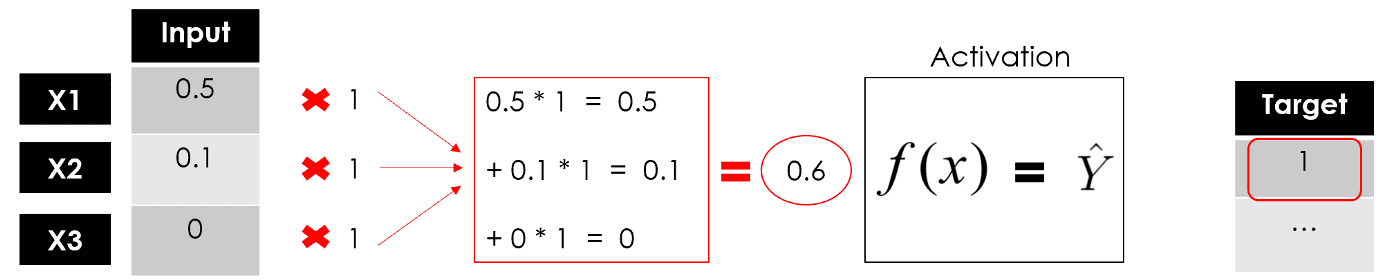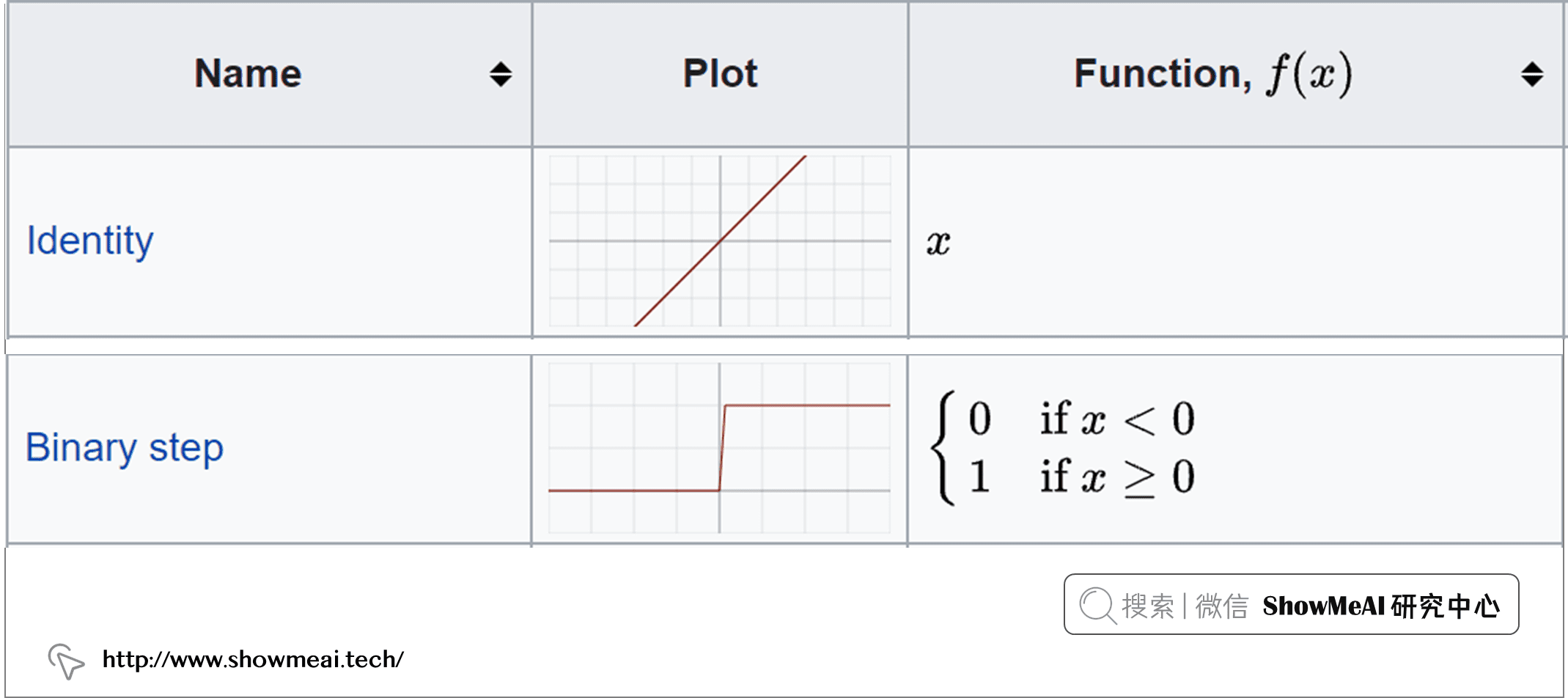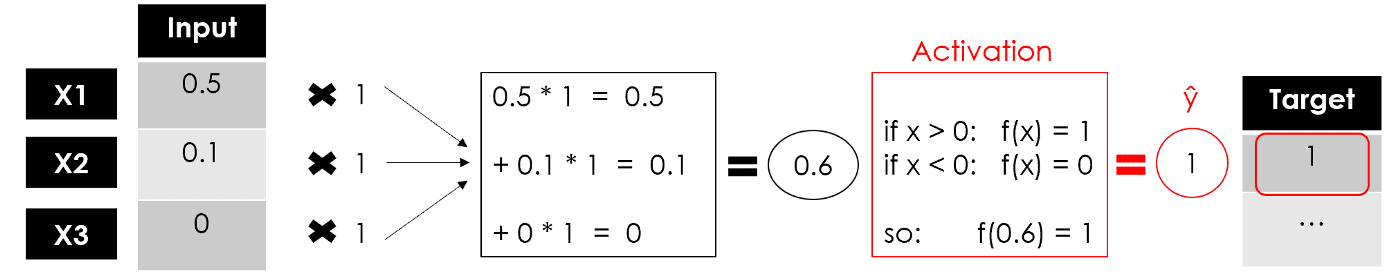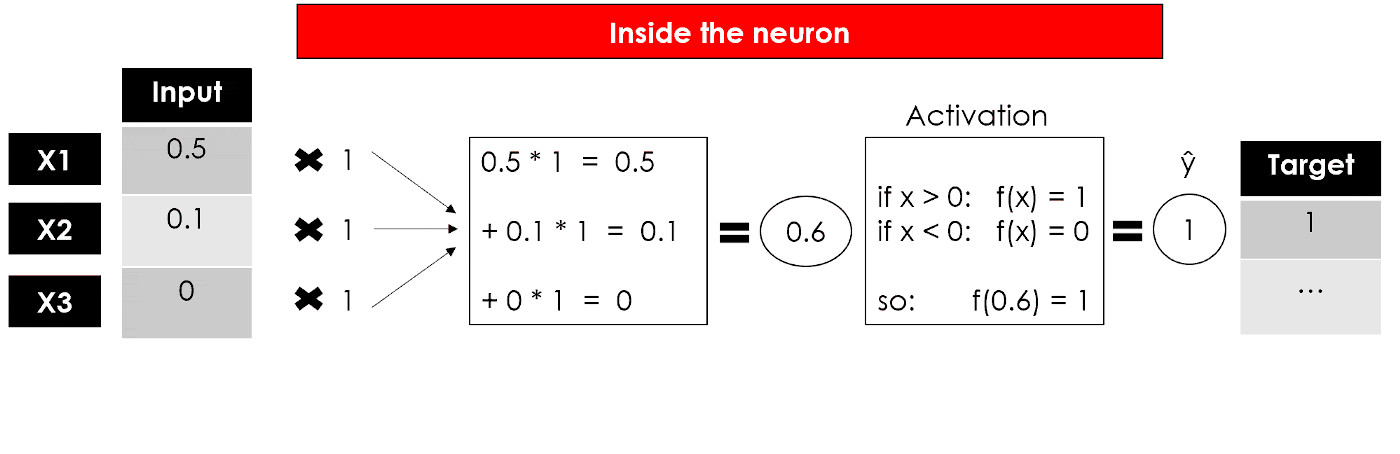# 💡 深度神经网络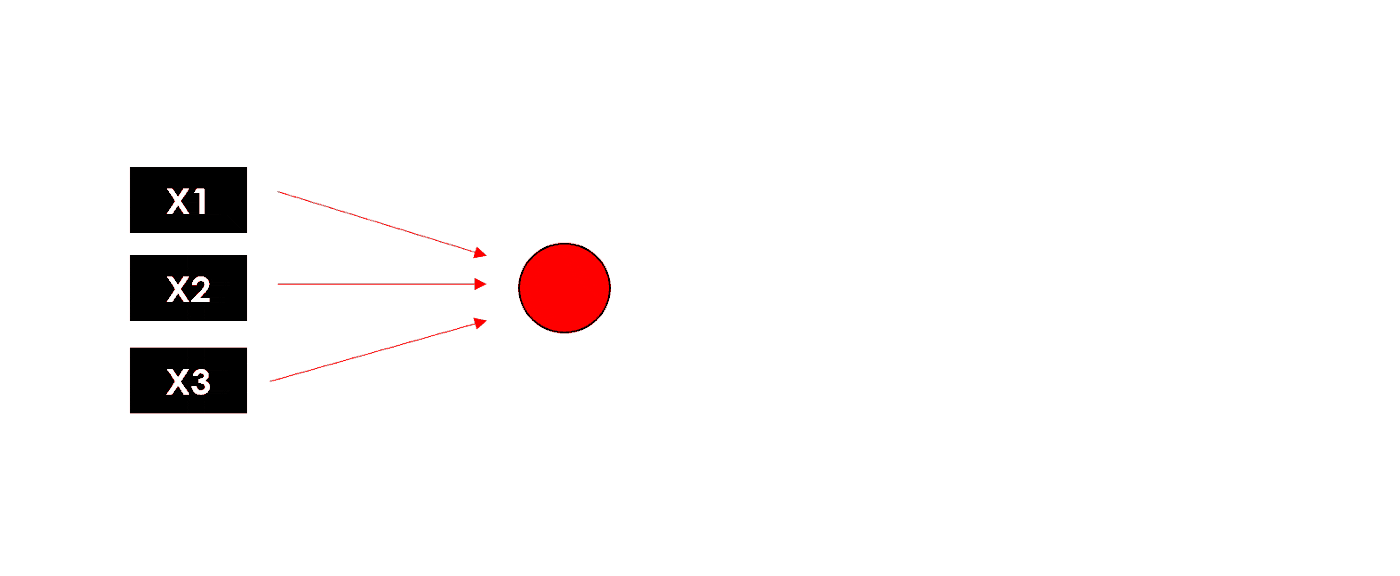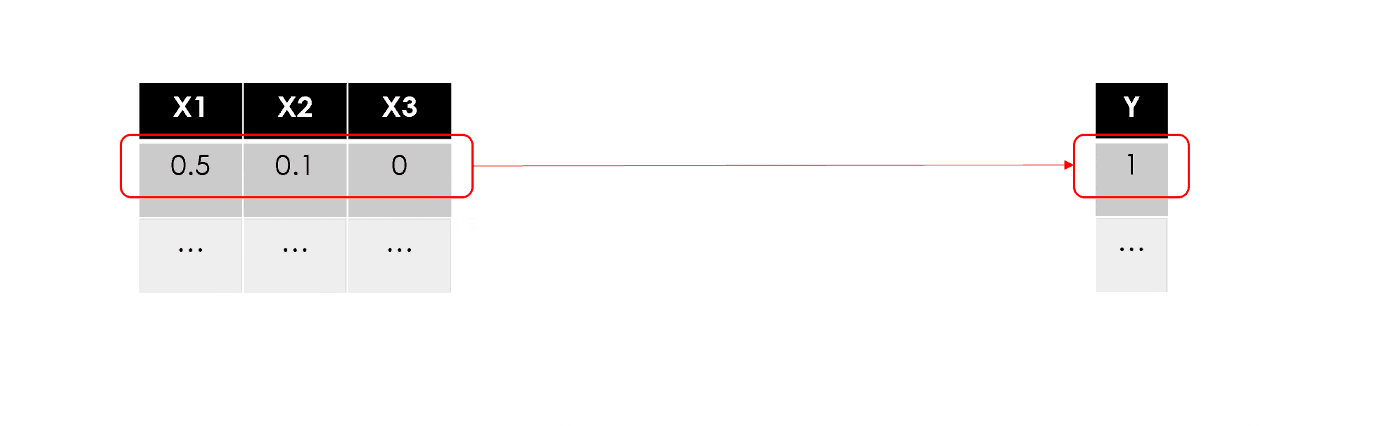• 偏置项bias：在每个神经元内部，输入和权重的线性组合也包括一个偏差，类似于线性方程中的常数，因此神经元的完整公式是

![](https://p3-juejin.byteimg.com/tos-cn-i-k3u1fbpfcp/5863fdcf08fe4020afec88162f6cc684~tplv-k3u1fbpfcp-zoom-1.image" width="40%" referrerpolicy="no-referrer">

• 反向传播：在训练期间，模型通过将误差传播回节点并更新参数（权重和偏差）来学习以最小化损失。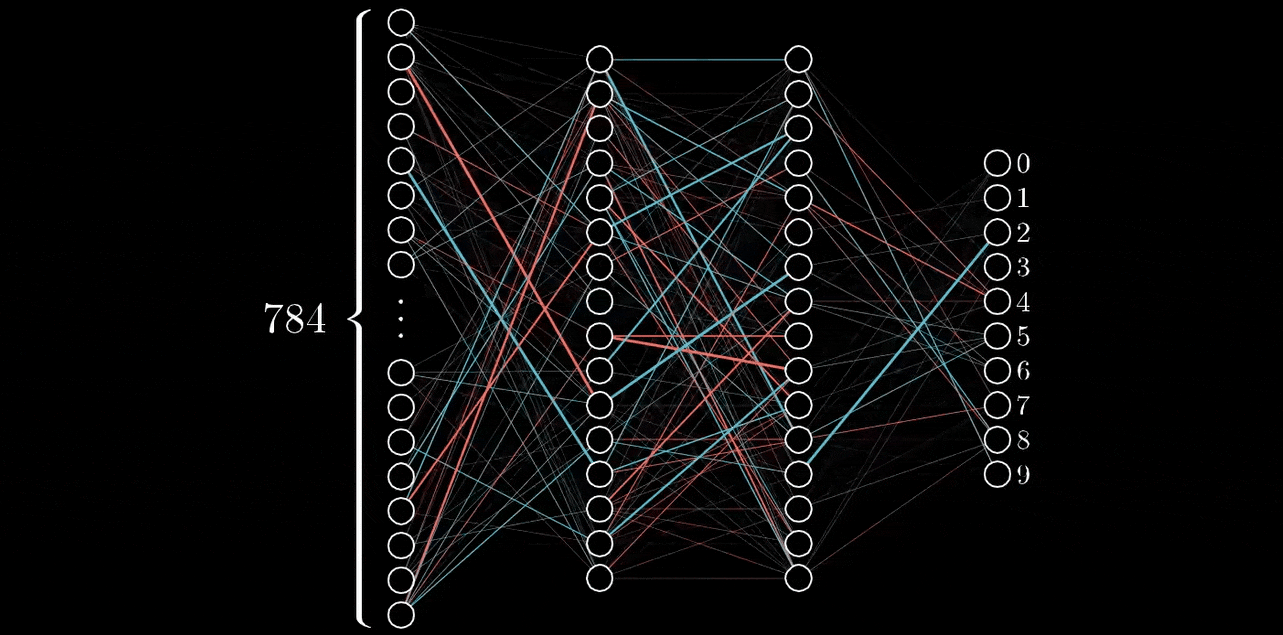• 梯度下降：用于训练神经网络的优化算法，通过在最陡下降方向上重复步骤来找到损失函数的局部最小值。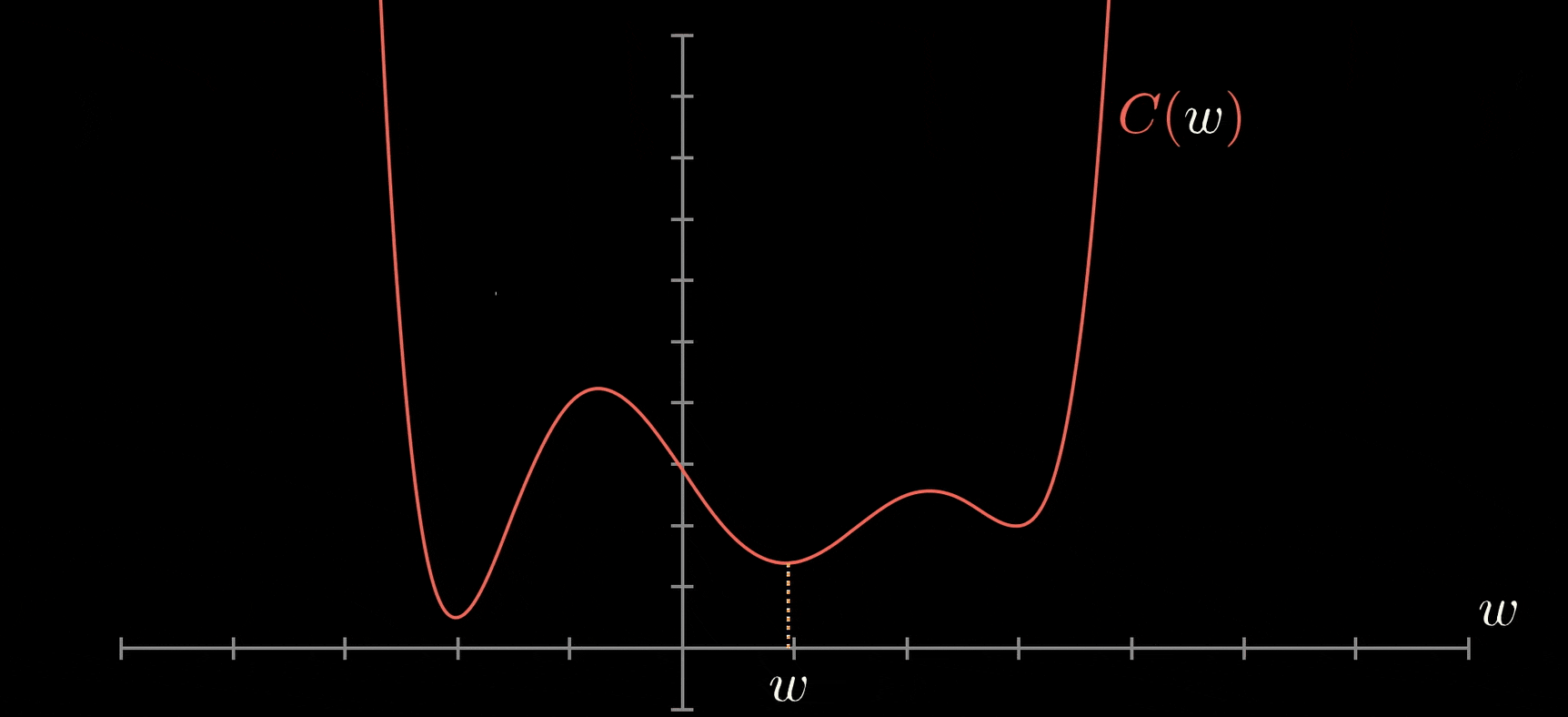# 💡 模型搭建

ShowMeAI制作了快捷即查即用的 Tensorflow 工具速查表手册，大家可以在下述位置获得：

model = models.Sequential(name="Perceptron",
layers=[
layers.Dense(             # 全连接层
name="dense",
input_dim=3,        # 输入维度为3
units=1,            # 1个节点
activation='linear' # 激活函数（这里是线性函数）
)
])
model.summary()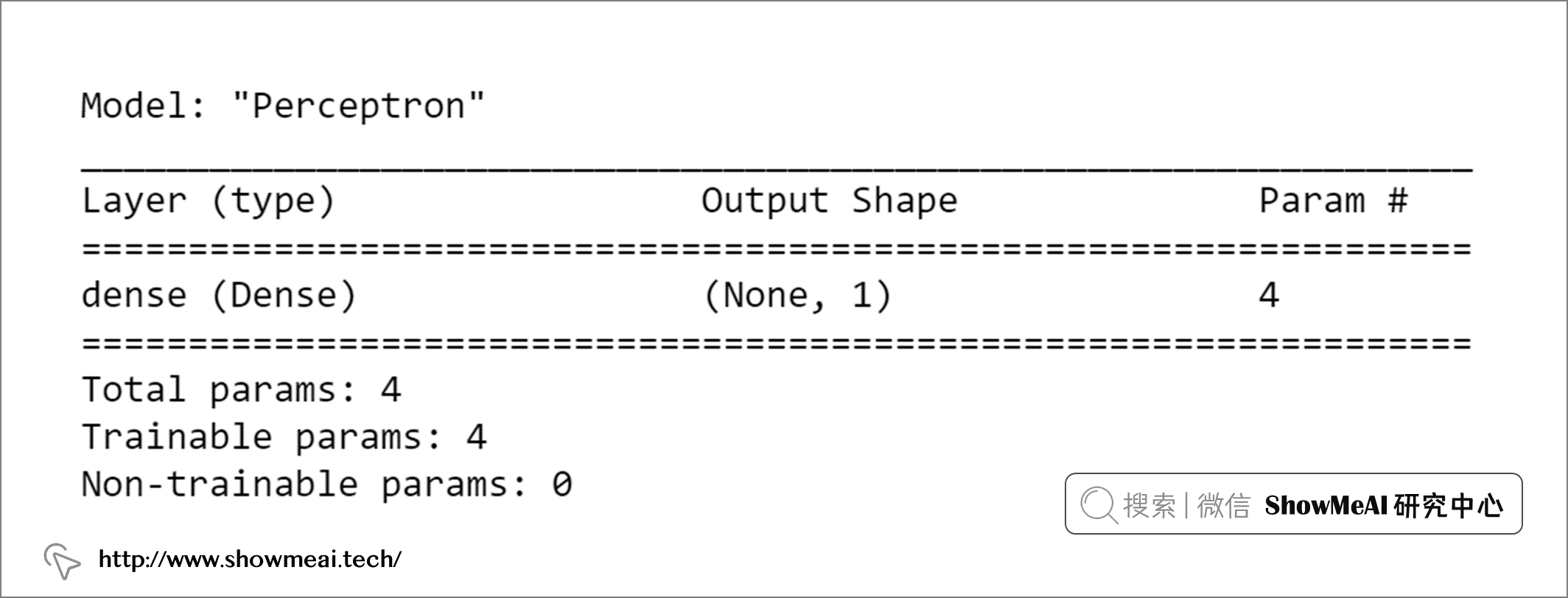model.summary操作可以输出网络的结构和参数等信息。当前情况下，我们只有 4 个（3 个权重和 1 个偏置项），所以它非常精简。

import tensorflow as tf
# 定义激活函数
def binary_step_activation(x):
# 如果x>0返回1否则返回0
return K.switch(x>0, tf.math.divide(x,x), tf.math.multiply(x,0))

# 构建模型
model = models.Sequential(name="Perceptron", layers=[
layers.Dense(
name="dense",
input_dim=3,
units=1,
activation=binary_step_activation
)
])

• 这是一个没有标准答案的问题，隐层的加入对于模型的学习能力提升有帮助，可以拟合更复杂的非线性情况，但也可能会导致过拟合（当然我们可以通过 Dropout 等技术来缓解过拟合，关于 Dropout，大家可以阅读学习ShowMeAI的文章 📘 深度学习教程 | 深度学习的实用层面
• 一般来说，简单的问题我们会用很少的层数（不超过3个隐层），复杂的问题我们使用的层数更多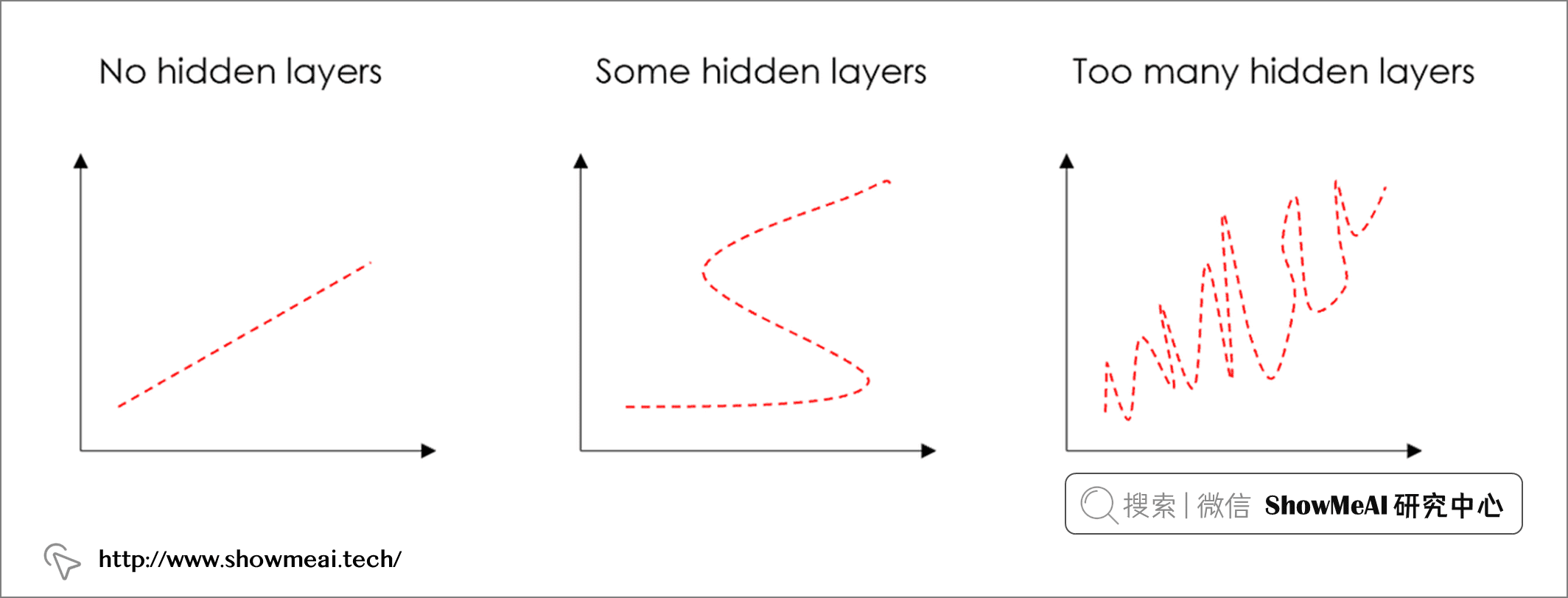• 这个有不同的设置方法，一个常用的设置方式是 (输入维度 + 输出维度)/2

• 激活函数有很多选择，其中没有哪一个一定好于另外一个。最常用的是 ReLU ，一个分段线性函数，仅在输出为正时才返回。
• 注意，在输出层必须具有与任务输出兼容的激活。例如，linear函数适用于回归问题，而 Sigmoid/softmax 经常用于分类。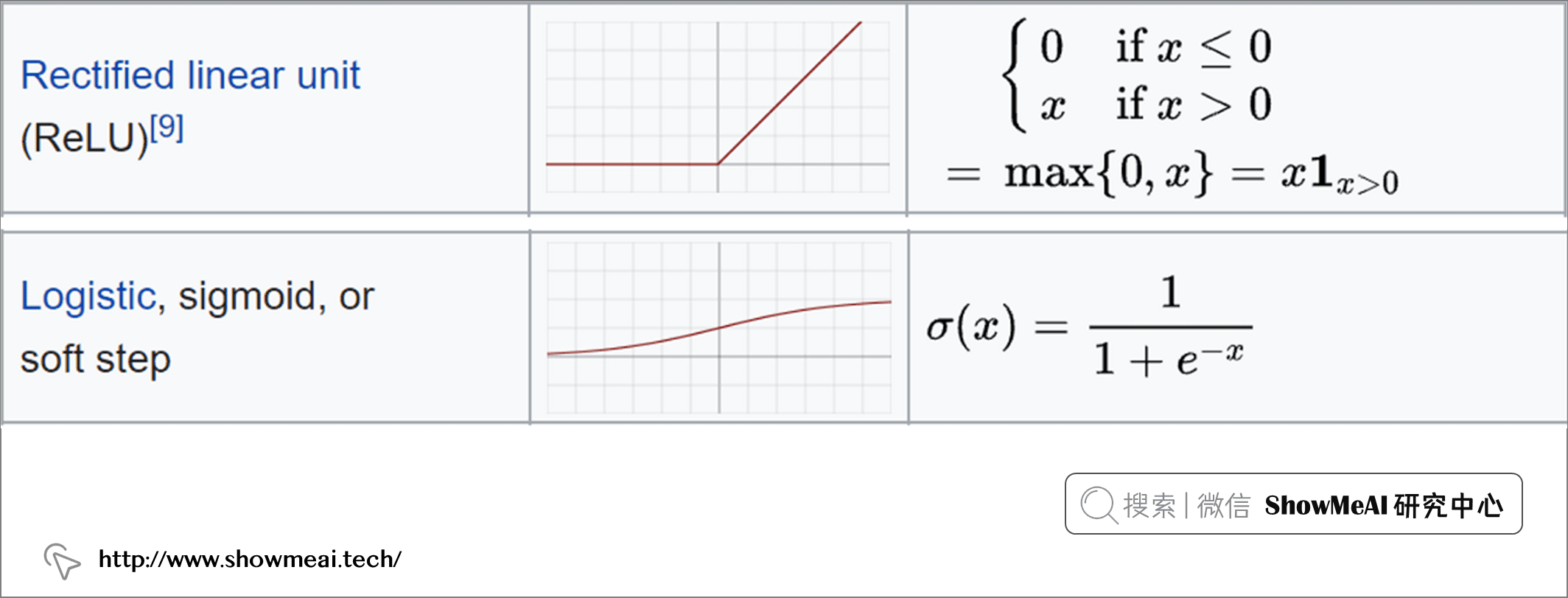n_features = 10

model = models.Sequential(name="DeepNN", layers=[
# 第1个隐层
layers.Dense(name="h1", input_dim=n_features,
units=int(round((n_features+1)/2)),
activation='relu'),
layers.Dropout(name="drop1", rate=0.2),

# 第2个隐层
layers.Dense(name="h2", units=int(round((n_features+1)/4)),
activation='relu'),
layers.Dropout(name="drop2", rate=0.2),

# 第3层
layers.Dense(name="output", units=1, activation='sigmoid')
])
model.summary()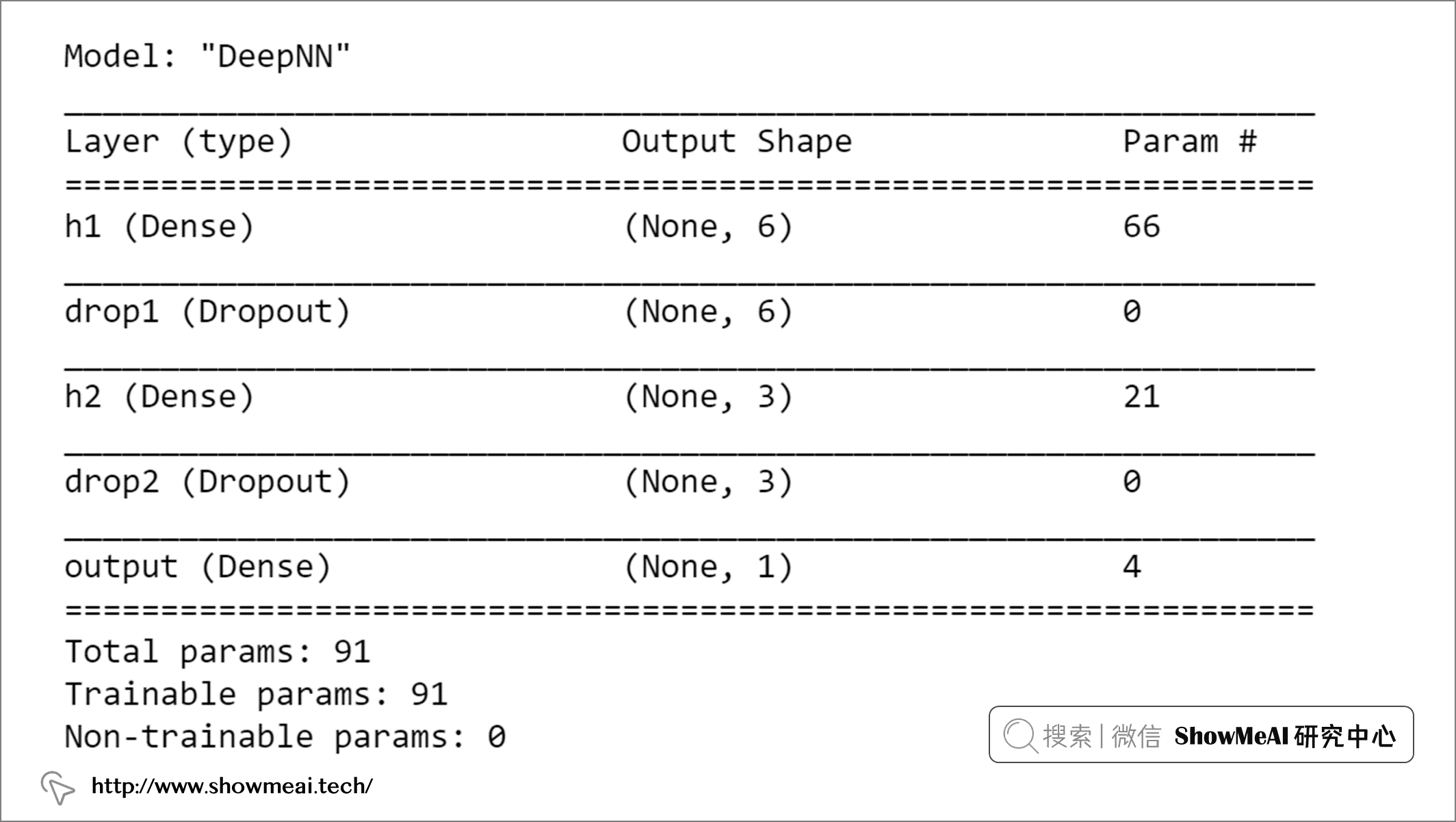• 需要指定输入层，而在 Sequential 类中它隐含在第一个 Dense 层的输入维度中。
• 每一层可以直接应用于其他层的输出，形如： output = layer(...)(input)

# 感知器
inputs = layers.Input(name="input", shape=(3,))
outputs = layers.Dense(name="output", units=1,
activation='linear')(inputs)
model = models.Model(inputs=inputs, outputs=outputs,
name="Perceptron")

# 深层神经网络
# 输入层
inputs = layers.Input(name="input", shape=(n_features,))

# 隐层1
h1 = layers.Dense(name="h1", units=int(round((n_features+1)/2)), activation='relu')(inputs)
h1 = layers.Dropout(name="drop1", rate=0.2)(h1)

# 隐层2
h2 = layers.Dense(name="h2", units=int(round((n_features+1)/4)), activation='relu')(h1)
h2 = layers.Dropout(name="drop2", rate=0.2)(h2)

# 输出层
outputs = layers.Dense(name="output", units=1, activation='sigmoid')(h2)

# 完整的模型
model = models.Model(inputs=inputs, outputs=outputs, name="DeepNN")

# 💡 神经网络结构可视化

'''

'''
def utils_nn_config(model):
lst_layers = []
if "Sequential" in str(model): #-> Sequential不显示输入层
layer = model.layers
lst_layers.append({"name":"input", "in":int(layer.input.shape[-1]), "neurons":0,
"out":int(layer.input.shape[-1]), "activation":None,
"params":0, "bias":0})
for layer in model.layers:
try:
dic_layer = {"name":layer.name, "in":int(layer.input.shape[-1]), "neurons":layer.units,
"out":int(layer.output.shape[-1]), "activation":layer.get_config()["activation"],
"params":layer.get_weights(), "bias":layer.get_weights()}
except:
dic_layer = {"name":layer.name, "in":int(layer.input.shape[-1]), "neurons":0,
"out":int(layer.output.shape[-1]), "activation":None,
"params":0, "bias":0}
lst_layers.append(dic_layer)
return lst_layers

'''

'''
def visualize_nn(model, description=False, figsize=(10,8)):
# 获取层次信息
lst_layers = utils_nn_config(model)
layer_sizes = [layer["out"] for layer in lst_layers]

# 绘图设置
fig = plt.figure(figsize=figsize)
ax = fig.gca()
ax.set(title=model.name)
ax.axis('off')
left, right, bottom, top = 0.1, 0.9, 0.1, 0.9
x_space = (right-left) / float(len(layer_sizes)-1)
y_space = (top-bottom) / float(max(layer_sizes))
p = 0.025

# 中间节点
for i,n in enumerate(layer_sizes):
top_on_layer = y_space*(n-1)/2.0 + (top+bottom)/2.0
layer = lst_layers[i]
color = "green" if i in [0, len(layer_sizes)-1] else "blue"
color = "red" if (layer['neurons'] == 0) and (i > 0) else color

## 添加信息说明
if (description is True):
d = i if i == 0 else i-0.5
if layer['activation'] is None:
plt.text(x=left+d*x_space, y=top, fontsize=10, color=color, s=layer["name"].upper())
else:
plt.text(x=left+d*x_space, y=top, fontsize=10, color=color, s=layer["name"].upper())
plt.text(x=left+d*x_space, y=top-p, fontsize=10, color=color, s=layer['activation']+" (")
plt.text(x=left+d*x_space, y=top-2*p, fontsize=10, color=color, s="Σ"+str(layer['in'])+"[X*w]+b")
out = " Y"  if i == len(layer_sizes)-1 else " out"
plt.text(x=left+d*x_space, y=top-3*p, fontsize=10, color=color, s=") = "+str(layer['neurons'])+out)

## 遍历
for m in range(n):
color = "limegreen" if color == "green" else color
circle = plt.Circle(xy=(left+i*x_space, top_on_layer-m*y_space-4*p), radius=y_space/4.0, color=color, ec='k', zorder=4)

## 添加文本说明
if i == 0:
plt.text(x=left-4*p, y=top_on_layer-m*y_space-4*p, fontsize=10, s=r'$X_{'+str(m+1)+'}$')
elif i == len(layer_sizes)-1:
plt.text(x=right+4*p, y=top_on_layer-m*y_space-4*p, fontsize=10, s=r'$y_{'+str(m+1)+'}$')
else:
plt.text(x=left+i*x_space+p, y=top_on_layer-m*y_space+(y_space/8.+0.01*y_space)-4*p, fontsize=10, s=r'$H_{'+str(m+1)+'}$')

# 添加链接箭头等
for i, (n_a, n_b) in enumerate(zip(layer_sizes[:-1], layer_sizes[1:])):
layer = lst_layers[i+1]
color = "green" if i == len(layer_sizes)-2 else "blue"
color = "red" if layer['neurons'] == 0 else color
layer_top_a = y_space*(n_a-1)/2. + (top+bottom)/2. -4*p
layer_top_b = y_space*(n_b-1)/2. + (top+bottom)/2. -4*p
for m in range(n_a):
for o in range(n_b):
line = plt.Line2D([i*x_space+left, (i+1)*x_space+left],
[layer_top_a-m*y_space, layer_top_b-o*y_space],
c=color, alpha=0.5)
if layer['activation'] is None:
if o == m:
else:
plt.show()

visualize_nn(model, description=True, figsize=(10,8))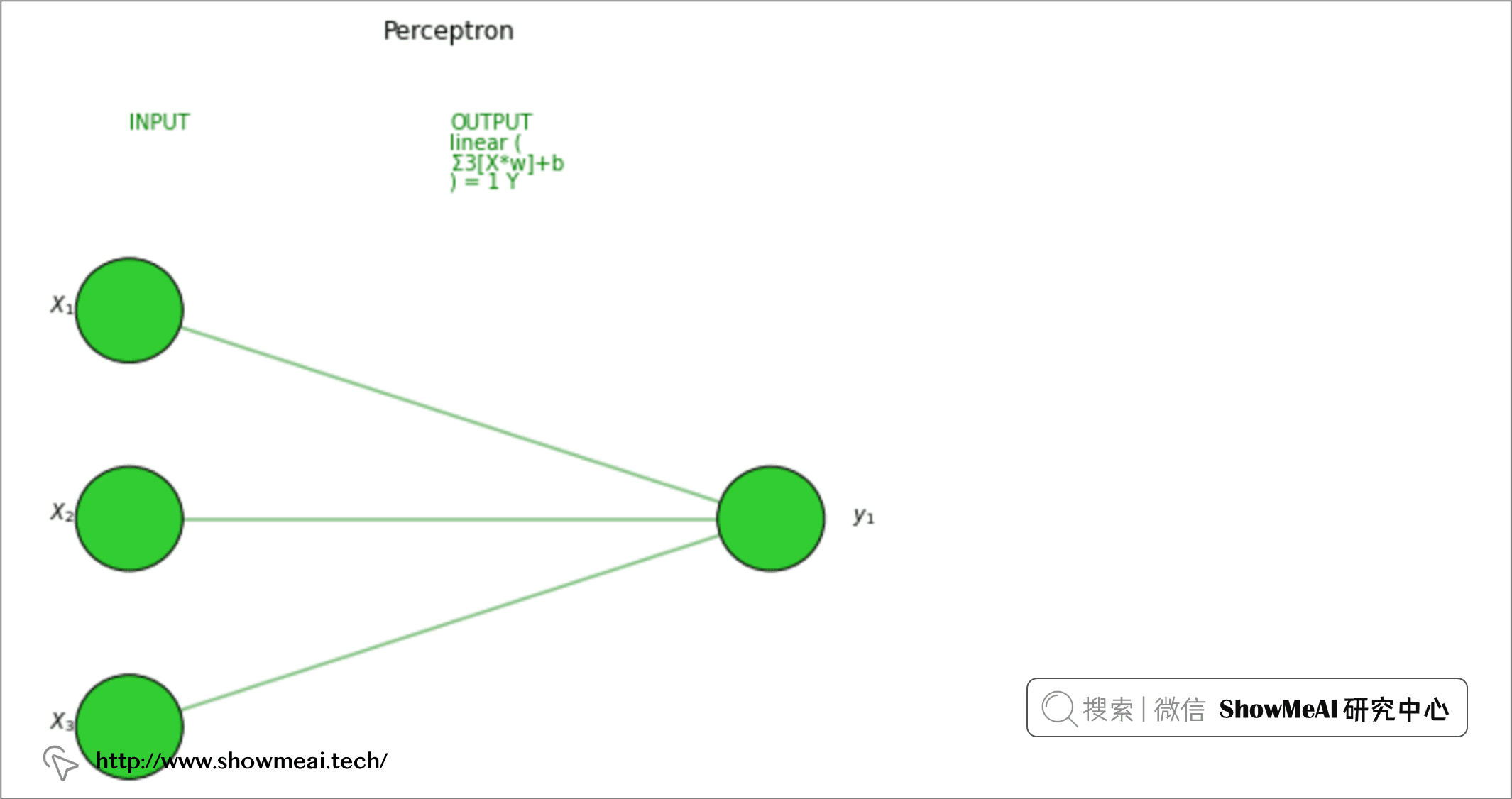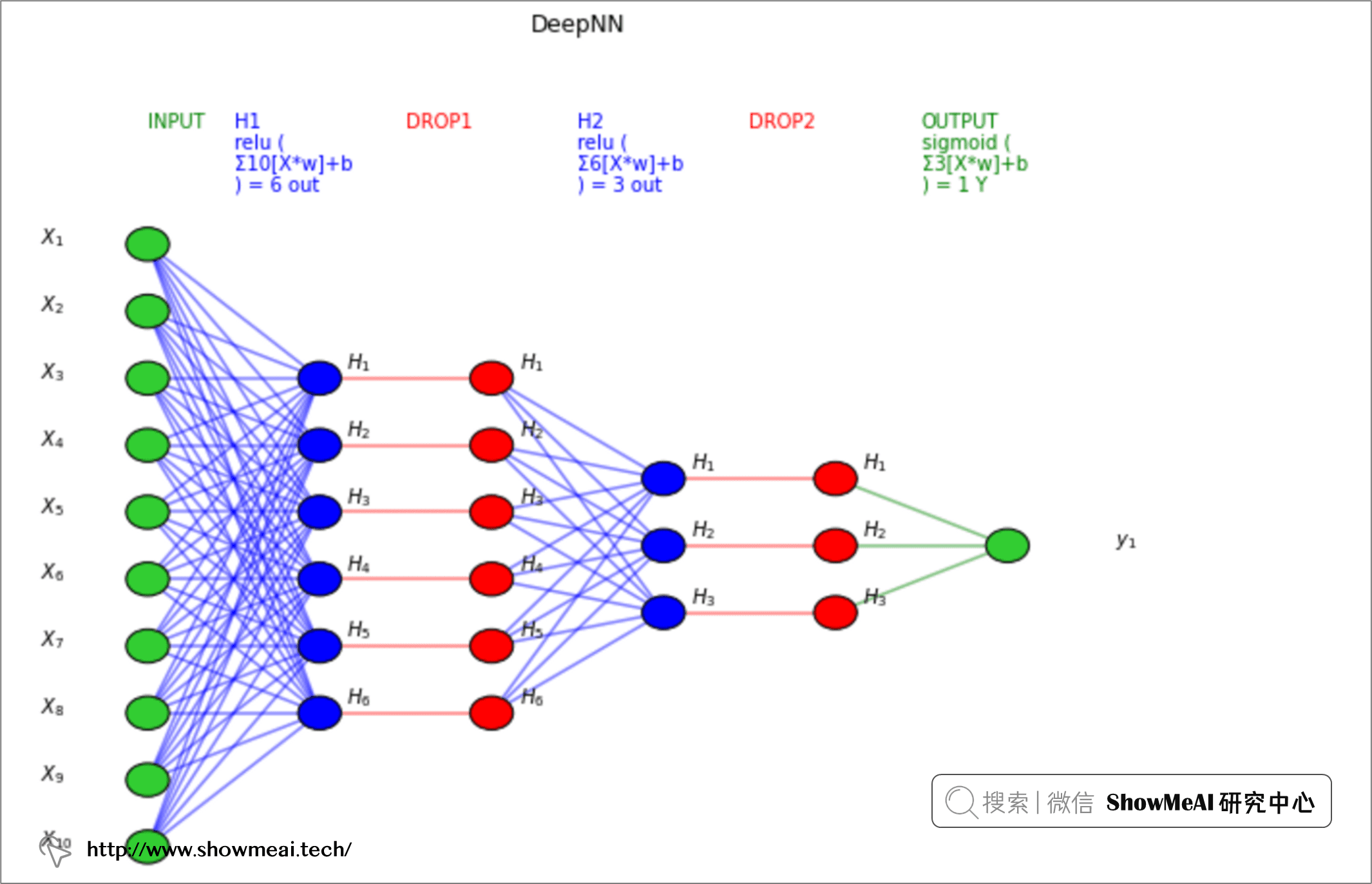utils.plot_model(model, to_file='model.png', show_shapes=True, show_layer_names=True)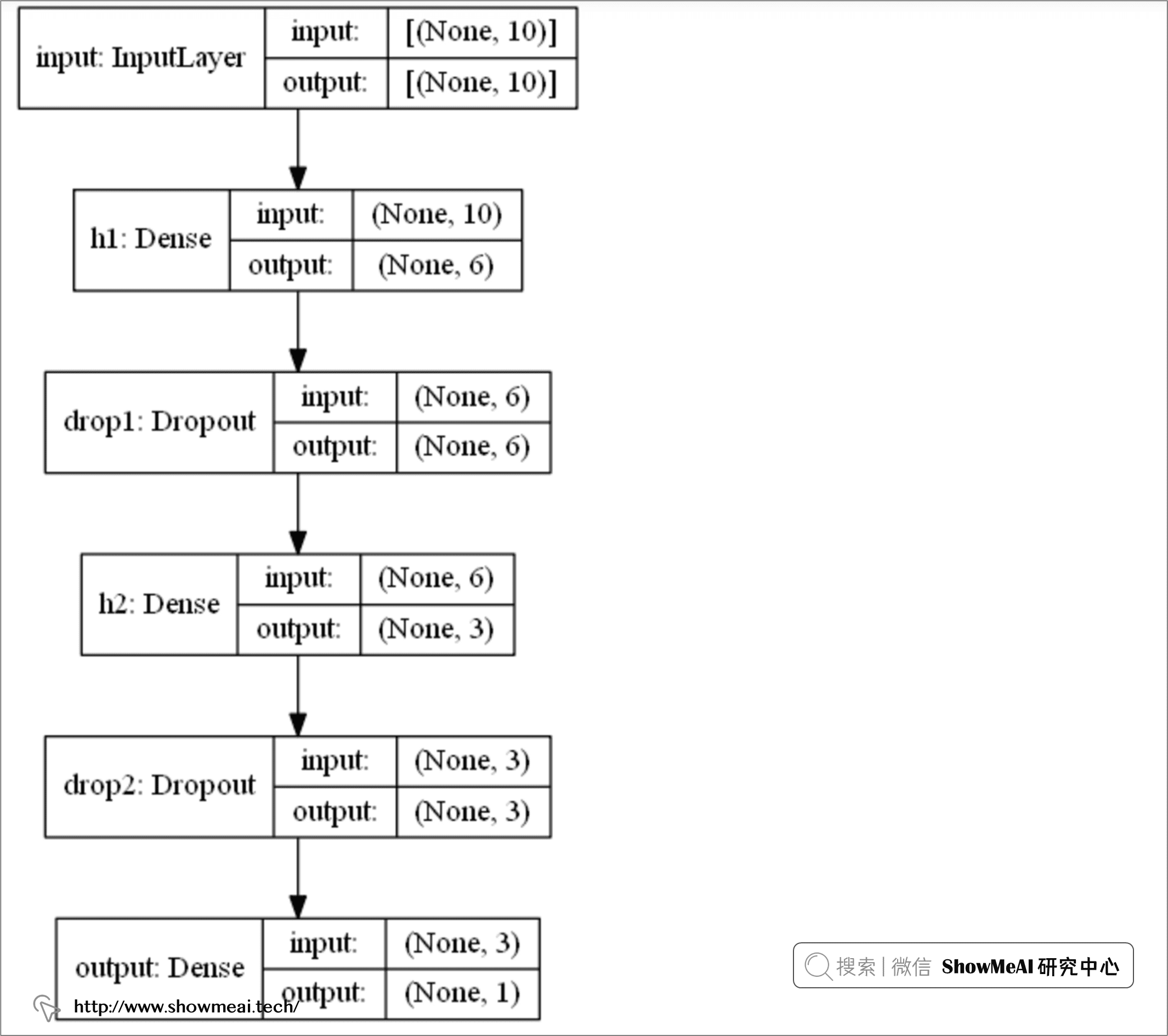# 💡 训练和测试评估

# 定义评估准则
def Recall(y_true, y_pred):
true_positives = K.sum(K.round(K.clip(y_true * y_pred, 0, 1)))
possible_positives = K.sum(K.round(K.clip(y_true, 0, 1)))
recall = true_positives / (possible_positives + K.epsilon())
return recall

def Precision(y_true, y_pred):
true_positives = K.sum(K.round(K.clip(y_true * y_pred, 0, 1)))
predicted_positives = K.sum(K.round(K.clip(y_pred, 0, 1)))
precision = true_positives / (predicted_positives + K.epsilon())
return precision

def F1(y_true, y_pred):
precision = Precision(y_true, y_pred)
recall = Recall(y_true, y_pred)
return 2*((precision*recall)/(precision+recall+K.epsilon()))

# 编译神经网络
metrics=['accuracy',F1]) 

# 定义R方评估准则
def R2(y, y_hat):
ss_res =  K.sum(K.square(y - y_hat))
ss_tot = K.sum(K.square(y - K.mean(y)))
return ( 1 - ss_res/(ss_tot + K.epsilon()) )

# 编译神经网络
metrics=[R2])

import numpy as np
X = np.random.rand(1000,10)
y = np.random.choice([1,0], size=1000)

# 训练和评估
training = model.fit(x=X, y=y, batch_size=32, epochs=100, shuffle=True, verbose=0, validation_split=0.2)

# 绘制评估指标
metrics = [k for k in training.history.keys() if ("loss" not in k) and ("val" not in k)]
fig, ax = plt.subplots(nrows=1, ncols=2, sharey=True, figsize=(15,3))

# 训练阶段
ax.set(title="Training")
ax11 = ax.twinx()
ax.plot(training.history['loss'], color='black')    ax.set_xlabel('Epochs')
ax.set_ylabel('Loss', color='black')
for metric in metrics:
ax11.plot(training.history[metric], label=metric)    ax11.set_ylabel("Score", color='steelblue')
ax11.legend()

# 验证集评估阶段
ax.set(title="Validation")
ax22 = ax.twinx()
ax.plot(training.history['val_loss'], color='black')    ax.set_xlabel('Epochs')
ax.set_ylabel('Loss', color='black')
for metric in metrics:
ax22.plot(training.history['val_'+metric], label=metric)    ax22.set_ylabel("Score", color="steelblue")
plt.show()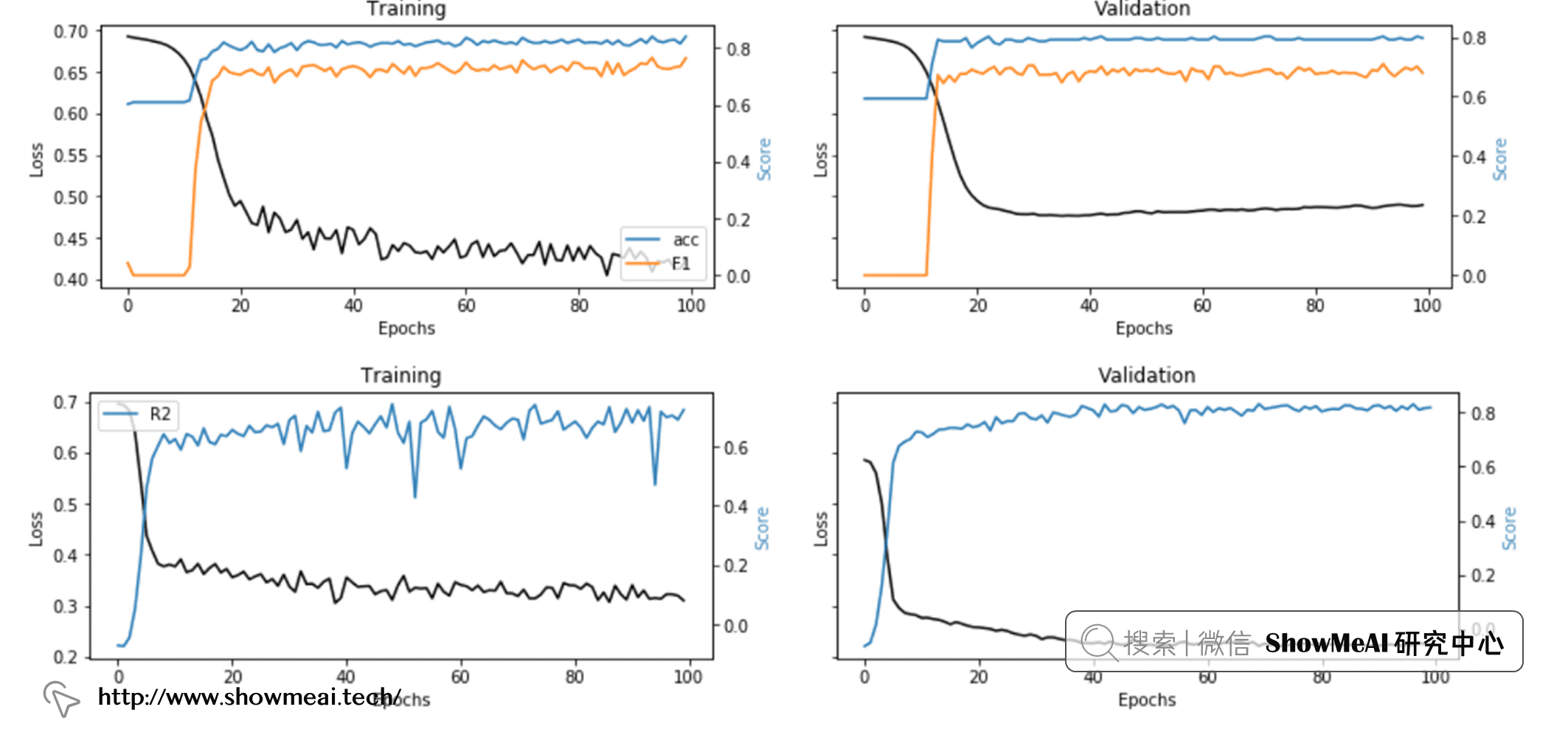# 💡 模型可解释性

'''

:parameter
:param model: model instance (after fitting)
:param X_names: list
:param X_instance: array of size n x 1 (n,)
:param X_train: array - if None the model is simple machine learning, if not None then it's a deep learning model
:param task: string - "classification", "regression"
:param top: num - top features to display
:return
dtf with explanations
'''
def explainer_shap(model, X_names, X_instance, X_train=None, task="classification", top=10):
# 构建解释器
# 机器学习（树模型）
if X_train is None:
explainer = shap.TreeExplainer(model)
shap_values = explainer.shap_values(X_instance)
# 深度学习（神经网络）
else:
explainer = shap.DeepExplainer(model, data=X_train[:100])
shap_values = explainer.shap_values(X_instance.reshape(1,-1)).reshape(-1)

# 绘图
# 分类场景
if task == "classification":
shap.decision_plot(explainer.expected_value, shap_values, link='logit', feature_order='importance',
features=X_instance, feature_names=X_names, feature_display_range=slice(-1,-top-1,-1))
# 回归场景
else:
shap.waterfall_plot(explainer.expected_value, shap_values,
features=X_instance, feature_names=X_names, max_display=top)

shap实际上是一个很有效的工具，大家在上述代码也可以看到，实际它可以应用在机器学习模型（如线性回归、随机森林）上，也可以应用在神经网络上。从代码中可以看出，如果 X_train 参数为 None，会选择机器学习模型进行解释，否则使用深度学习进行解释分析。

i = 1explainer_shap(model,
X_names=list_feature_names,
X_instance=X[i],
X_train=X,
top=10)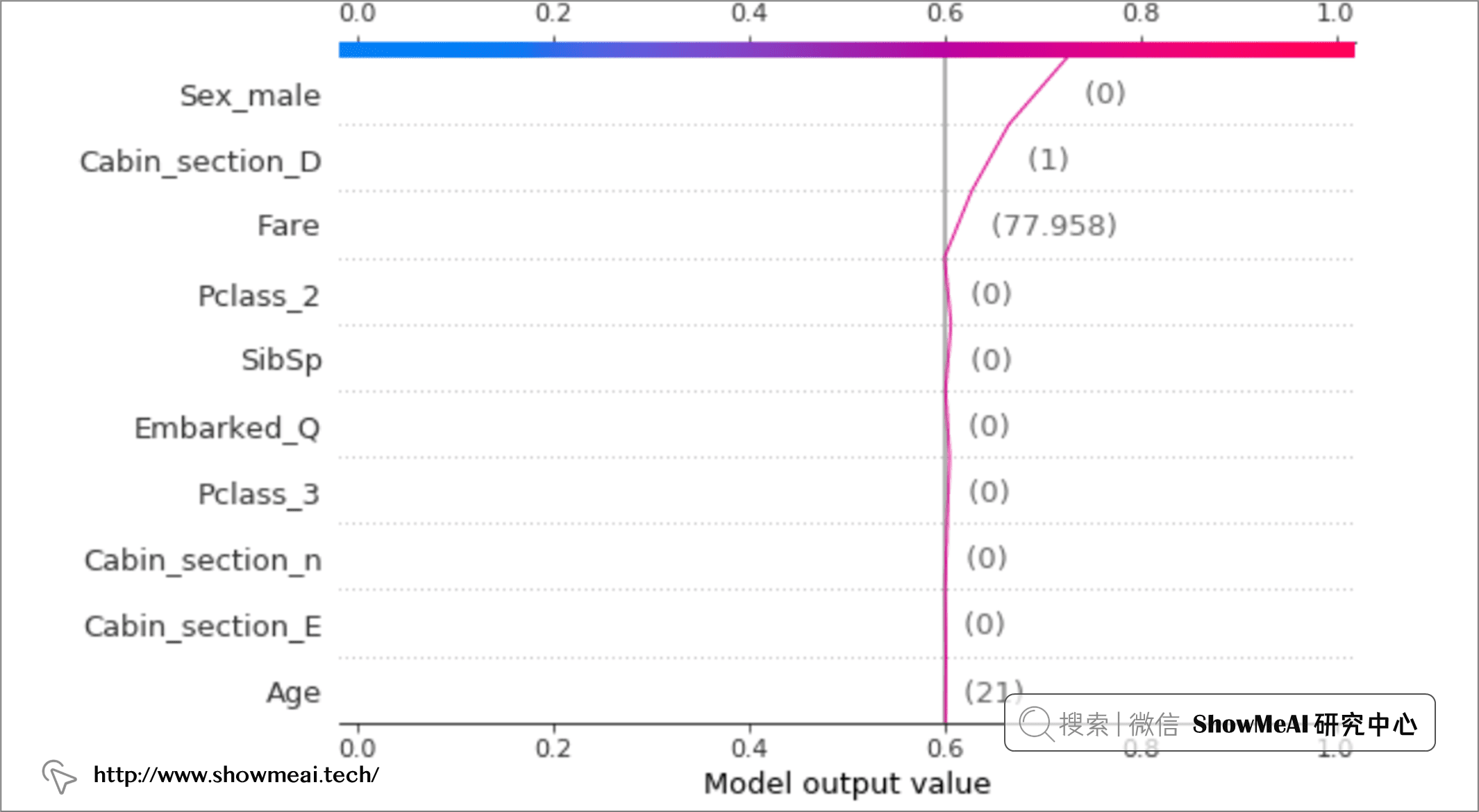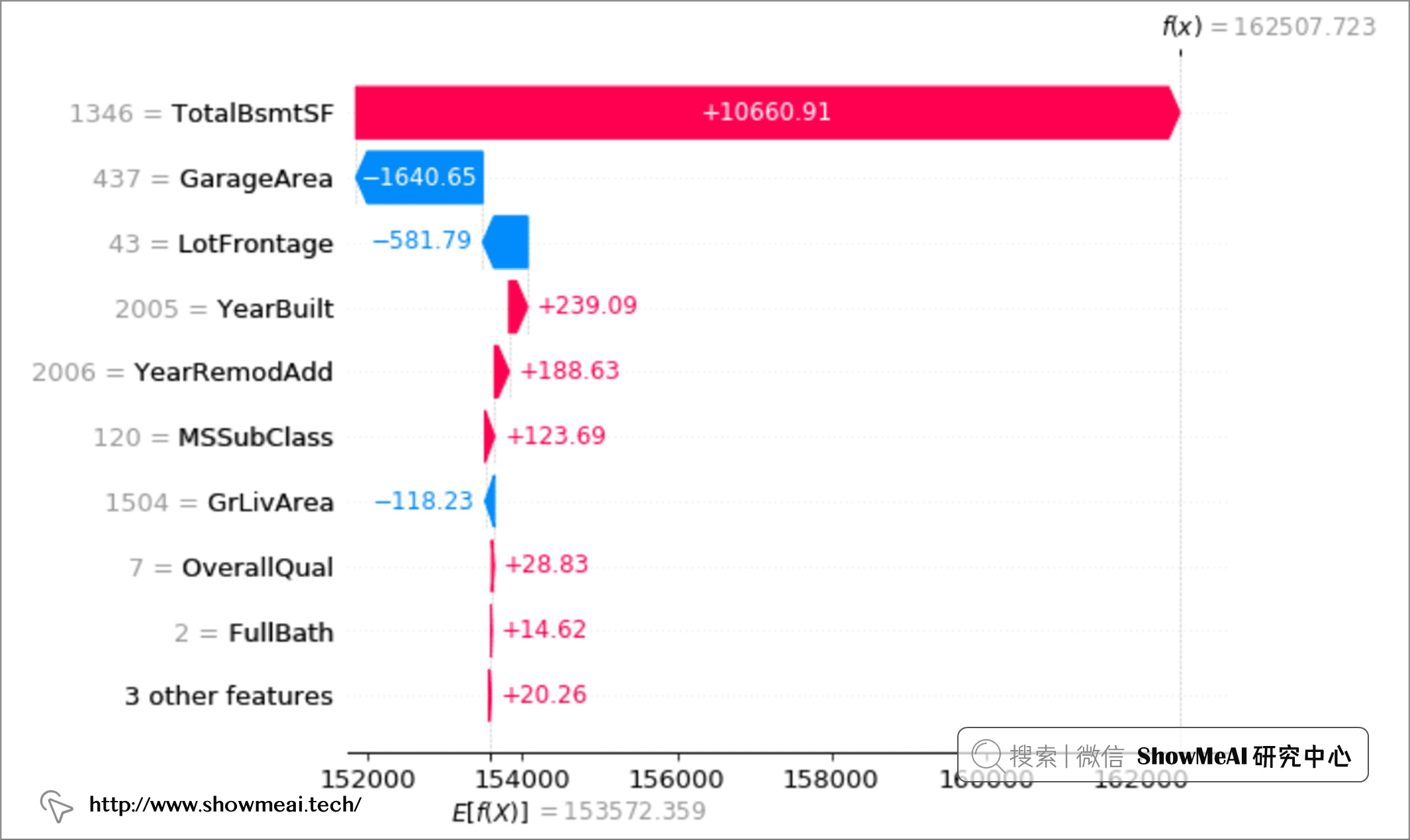# 参考资料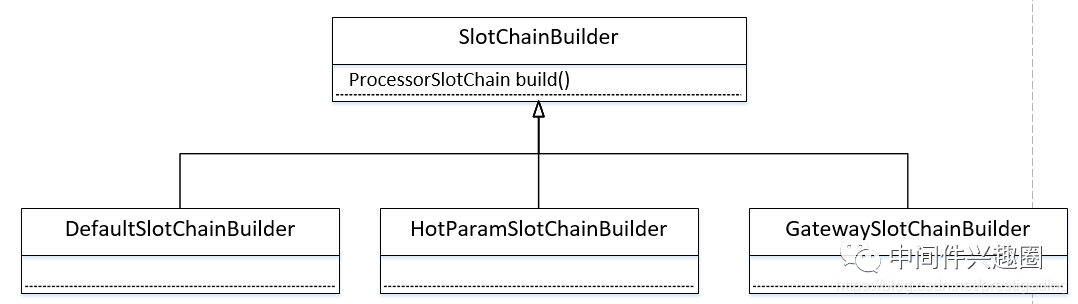30 0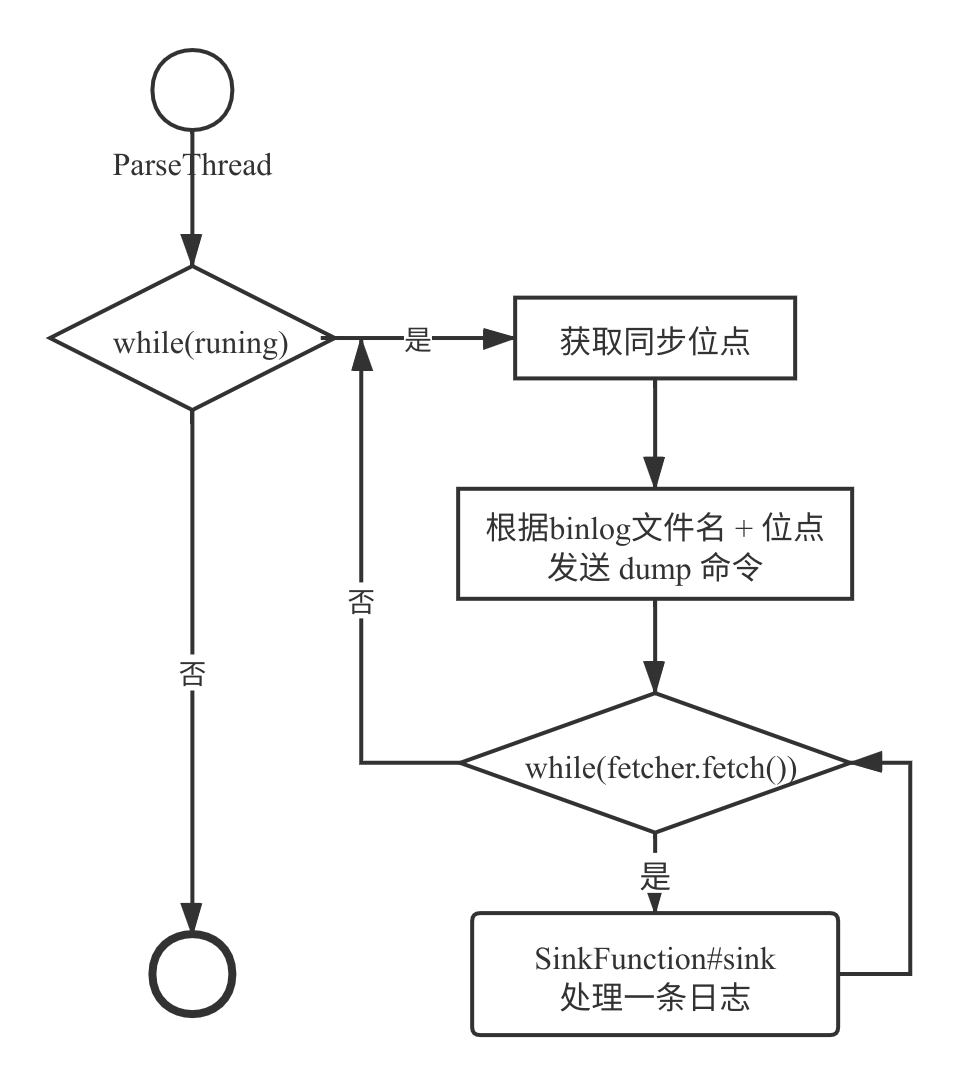Canal binlog 日志 Dump 流程分析
Canal binlog 日志 Dump 流程分析
118 0WinCE6.0 USB Fubction驱动加载流程
812 01084 0《中国人工智能学会通讯》——4.2 视频监控依然存在问题

1330 0js流程语句的复习
js流程语句分为单行语句和复合语句；用花括号包含的语句集合叫做复合语句，一对花括号表示一个复合语句，处理的时候可以当作一条单行语句来对待，复合语句一般也被称为代码块
1461 0OAuth的机制原理讲解及开发流程

1004 0《夜思香》——献给编程他乡的同志们
夜思香 风言风语风雨中 一花一叶一夜空 不安蜀山长江水 那山那水那不同
624 0+关注
ShowMeAI
『为AI硬核资料库(cool)而生！』构建AI解决方案，用知识加速每一次技术成长！网站：https://www.showmeai.tech/ 公众号：ShowMeAI研究中心
193

0